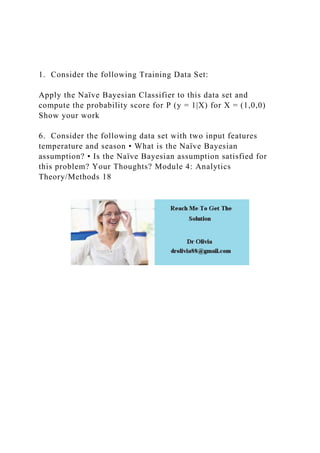Seu SlideShare está sendo baixado. ×

# 1.  Consider the following Training Data SetApply the Naïve.docx

Anúncio
Anúncio
Anúncio
Anúncio
Anúncio
Anúncio
Anúncio
Anúncio
Anúncio
Anúncio
AnúncioCarregando em…3
×

1 de 1 Anúncio

# 1.  Consider the following Training Data SetApply the Naïve.docx

1.  Consider the following Training Data Set:

Apply the Naïve Bayesian Classifier to this data set and compute the probability score for P (y = 1|X) for X = (1,0,0) Show your work

6.  Consider the following data set with two input features temperature and season • What is the Naïve Bayesian assumption? • Is the Naïve Bayesian assumption satisfied for this problem? Your Thoughts? Module 4: Analytics Theory/Methods 18
.

1.  Consider the following Training Data Set:

Apply the Naïve Bayesian Classifier to this data set and compute the probability score for P (y = 1|X) for X = (1,0,0) Show your work

6.  Consider the following data set with two input features temperature and season • What is the Naïve Bayesian assumption? • Is the Naïve Bayesian assumption satisfied for this problem? Your Thoughts? Module 4: Analytics Theory/Methods 18
.

Anúncio
Anúncio

### 1.  Consider the following Training Data SetApply the Naïve.docx

1. 1. 1. Consider the following Training Data Set: Apply the Naïve Bayesian Classifier to this data set and compute the probability score for P (y = 1|X) for X = (1,0,0) Show your work 6. Consider the following data set with two input features temperature and season • What is the Naïve Bayesian assumption? • Is the Naïve Bayesian assumption satisfied for this problem? Your Thoughts? Module 4: Analytics Theory/Methods 18